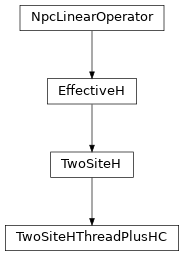Inheritance DiagramMethods

 `TwoSiteHThreadPlusHC.__init__`(*args[, ...]) Return the hermitian conjugate of self. `TwoSiteHThreadPlusHC.combine_Heff`(env[, ...]) Combine LP and RP with W to form LHeff and RHeff. Combine the legs of theta, such that it fits to how we combined the legs of self. Apply the effective Hamiltonian to theta. `TwoSiteHThreadPlusHC.matvec_hc`(theta) Contract self to a matrix. `TwoSiteHThreadPlusHC.update_LP`(env, i[, U]) Equivalent to `env.get_LP(i, store=True)`; optimized for combine. `TwoSiteHThreadPlusHC.update_RP`(env, i[, VH]) Equivalent to `env.get_RP(i, store=True)`; optimized for combine.

Class Attributes and Properties

 `TwoSiteHThreadPlusHC.acts_on` `TwoSiteHThreadPlusHC.length`

Bases: `TwoSiteH`

Version of TwoSiteH that parallelizes matvec with threads.

Using threads instead of e.g. MPI parallelization means we don’t need to make explicit copies of (at least one of) the environment tensors and communication is much cheaper.

matvec(theta)[source]

Apply the effective Hamiltonian to theta.

Parameters

theta (`Array`) – Labels: `vL, p0, p1, vR` if combine=False, `(vL.p0), (p1.vR)` if True

Returns

Product of theta and the effective Hamiltonian.

Return type

theta `Array`

to_matrix()[source]

Contract self to a matrix.

Return the hermitian conjugate of self.

combine_Heff(env, left=True, right=True)[source]

Combine LP and RP with W to form LHeff and RHeff.

Combine LP with W0 and RP with W1 to get the effective parts of the Hamiltonian with piped legs.

Parameters
• env (`MPOEnvironment`) – Environment for contraction `<psi|H|psi>`.

• left (bool) – The mixer might need only one of LHeff/RHeff after the Lanczos optimization even for combine=True. These flags allow to calculate them specifically.

• right (bool) – The mixer might need only one of LHeff/RHeff after the Lanczos optimization even for combine=True. These flags allow to calculate them specifically.

combine_theta(theta)[source]

Combine the legs of theta, such that it fits to how we combined the legs of self.

Parameters

theta (`Array`) – Wave function with labels `'vL', 'p0', 'p1', 'vR'`

Returns

theta – Wave function with labels `'vL', 'p0', 'p1', 'vR'`

Return type

`Array`

update_LP(env, i, U=None)[source]

Equivalent to `env.get_LP(i, store=True)`; optimized for combine.

Parameters
• env (`MPOEnvironment`) – The same environment as given during class initialization.

• i (int) – We update the part left of site i. Can optimize if i == `i0` and `combine` is True.

• U (None | `Array`) – The tensor on the left-most site self acts on, with combined legs after SVD. Only used if trying to optimize.

update_RP(env, i, VH=None)[source]

Equivalent to `env.get_RP(i, store=True)`; optimized for combine.

Parameters
• env (`MPOEnvironment`) – The same environment as given during class initialization.

• i (int) – We update the part right of site i. Can optimize if i == `i0` + 2 - `length` and `combine` is True.

• U (None | `Array`) – The tensor on the right-most site self acts on, with combined legs after SVD. Only used if trying to optimize.